Courses

# Test: Strength Of Material (Level -II)- 1

## 25 Questions MCQ Test Mechanical Engineering SSC JE (Technical) | Test: Strength Of Material (Level -II)- 1

Description
This mock test of Test: Strength Of Material (Level -II)- 1 for Mechanical Engineering helps you for every Mechanical Engineering entrance exam. This contains 25 Multiple Choice Questions for Mechanical Engineering Test: Strength Of Material (Level -II)- 1 (mcq) to study with solutions a complete question bank. The solved questions answers in this Test: Strength Of Material (Level -II)- 1 quiz give you a good mix of easy questions and tough questions. Mechanical Engineering students definitely take this Test: Strength Of Material (Level -II)- 1 exercise for a better result in the exam. You can find other Test: Strength Of Material (Level -II)- 1 extra questions, long questions & short questions for Mechanical Engineering on EduRev as well by searching above.
QUESTION: 1

Solution:
QUESTION: 2

Solution:
QUESTION: 3

### The deformation of a bar under its own weight as compared to that when subjected to a direct axial load equal to its own weight will be

Solution:
QUESTION: 4

A slender bar of 100 mm2 cross-section is subjected to loading as shown in the figure below. If the modulus of elasticity is taken as 200 × 109. pa. then the elongation produced in the bar will be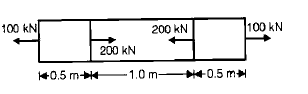Solution:
QUESTION: 5

A straight bar is fixed at edges A and B. its elastic modulus is E and cross-section is A. There is a load P = 120 N acting at C. The reactions at the ends are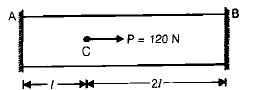Solution:
QUESTION: 6

In a tensile test, near the elastic limit zone

Solution:
QUESTION: 7

If Poisson's ratio of a material is 0.5, then the elastic modulus for the material is

Solution:
QUESTION: 8

The number of independent elastic constants required to express the stress strain relationship for a linearly elastic isotropic material is

Solution:
QUESTION: 9

The number of elastic constants for a completely anisotropic elastic material is

Solution:
QUESTION: 10

For a given material, the modulus of rigidity is 100 GPa and Poisson's ratio is 0.25. The value of modulus of elasticity in GPa is

Solution:
QUESTION: 11

The volumetric strain in case of a thin cylindrical shell of diameter 'd', thickness 't', subjected to  internal pressure 'P' is

Solution:
QUESTION: 12

The modulus of elasticity for a material is 200 GN/m2 and Poisson's ratio is 0.25. What is the modulus of rigidity ?

Solution:
QUESTION: 13

E, G, K andm represent the elastic modulus, shear modulus, Bulk modulus and poisson's ratio respectively of a linearly elastic. isotropic and homogeneous material. To express the stressstrain relations completely for this material, at  least

Solution:
QUESTION: 14

What is the relationship between the linear elastic properties; Young's modulus (E), rigidity modulus (G) and bulk modulus (K) ?

Solution:
QUESTION: 15

A copper rod 400 mm long is pulled in tension to a length of 401.2 mm by applying a tensile stress of 330 MPa. If the deformation is entirely elastic, the Young's Modulus of copper is

Solution:
QUESTION: 16

Match List – I (State of stress) with List -II (kind of loading) and select the correct answer using the codes given below the lists: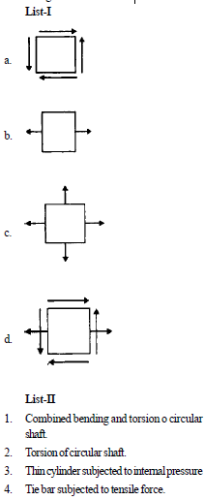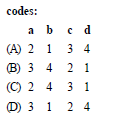Solution:
QUESTION: 17

State of stress at a point in a strained body is shown in figure A. which one of the figure given below represents correctly the mohr's circle for the state of stress?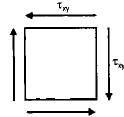Solution:
QUESTION: 18

Plane stress at a point in a body is defined by principal stresses 3s and s. The ratio of the normal stress to the maximum shear stress on the plane of maximum shear stress is

Solution:
QUESTION: 19

Which one of the following Mohr's circles represents the state of pure shear?

Solution:
QUESTION: 20

In a two dimensional problem the state of pure shear at a point is characterized by

Solution:
QUESTION: 21

A cantilever is loaded by a concentrated load P at the free and as shown. The shear stress in the element  LMNOPQRS is under consideration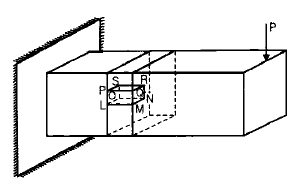Which of the following figures represents the shear stress direction in the cantilever ?

Solution:
QUESTION: 22

A circular shaft subjected to twisting moment results in maximum shear stress of 60 MPa. Then the maximum compressive stress in the material is

Solution:
QUESTION: 23

Consider a two dimensional state of stress given for an element as shown in the diagram given below: What are the coordinates of the centre of Mohr's circle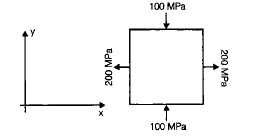Solution:
QUESTION: 24

At a point in two-dimensional stress system sx = 100 N/mm2,sy =txy= 400 N/mm2. what drawn with a scale of 1cm = 100 N/mm2.

Solution:
QUESTION: 25

A body is subjected to a pure tensile stress of 100 units. What is the maximum shear produced in the body at some oblique plane due to the above ?

Solution: